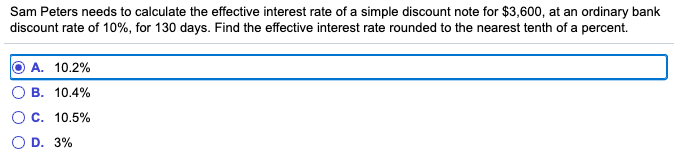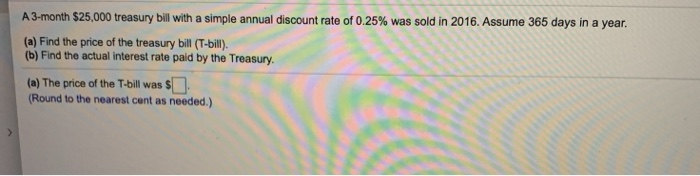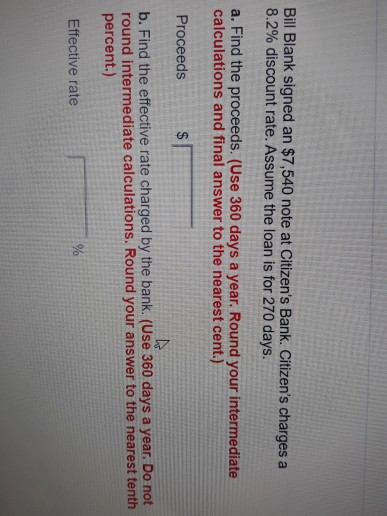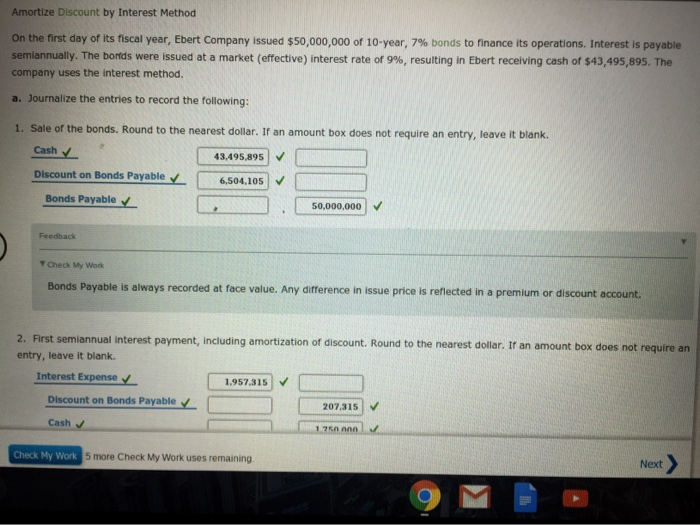Question
Find the actual interest paid, to the nearest tenth, on the simple discount note.
\$27,000; discount rate 7%; length of loan 3 mo.
\$50,000; discount rate 8%; length of loan 6 mo.

1) 21000*.07*.25=\$472.50 2) 50000*.08*.5=\$2000.00

#### Earn Coins

Coins can be redeemed for fabulous gifts.

Similar Homework Help Questions
• ### Please Help: Find the actual interest rate paid, to the nearest tenth, on the simple discount note

Please Help: Find the actual interest rate paid, to the nearest tenth, on the simple discount note. \$1000; discount rate 7.8%; length of loan 6 mo A) 10.1% B) 9.1% C) 7.1% D) 8.1%

• ### Sam Peters needs to calculate the effective interest rate of a simple discount note for \$3,600,...Sam Peters needs to calculate the effective interest rate of a simple discount note for \$3,600, at an ordinary bank discount rate of 10%, for 130 days. Find the effective interest rate rounded to the nearest tenth of a percent. O A. 10.2% OB. 10.4% O C. 10.5% OD. 3%

• ### Business math: simple interest question

You were offered either a simple interest note or a simple discount note with the following terms: \$33,264 at 8% for 18 months. Calculate the effective interest rate. (Do not round intermediate calculations. Round your final answer to the nearest tenth percent.) Please help I have been trying to do this problem for an hour and I can't find the correct answer

• ### Find the interest paid on a loan of \$2,200 for two years at a simple interest...

Find the interest paid on a loan of \$2,200 for two years at a simple interest rate of 11% per year. The interest on the loan is \$17

• ### A 3-month \$25,000 treasury bill with a simple annual discount rate of 0.25% was sold in...A 3-month \$25,000 treasury bill with a simple annual discount rate of 0.25% was sold in 2016. Assume 365 days in a year. (a) Find the price of the treasury bill (T-bill). (b) Find the actual interest rate paid by the Treasury. (a) The price of the T-bill was \$] (Round to the nearest cent as needed.) A 6-month \$20,500 treasury bill with a simple annual discount rate of 0.44% was sold in 2016. Assume 365 days in a year....

• ### Bill Blank signed an \$7,540 note at Citizen's Bank. Citizen's charges a 8.2% discount rate. Assume...Bill Blank signed an \$7,540 note at Citizen's Bank. Citizen's charges a 8.2% discount rate. Assume the loan is for 270 days. a. Find the proceeds. (Use 360 days a year. Round your intermediate calculations and final answer to the nearest cent.) Proceeds b. Find the effective rate charged by the bank. (Use 360 days a year. Do not round intermediate calculations. Round your answer to the nearest tenth percent.) Effective rate You were offered the opportunity to purchase either...

• ### Compound Interest and Simple Interest / Finite Math

I have a few problems I need help in Finate Math please help me :( I keep getting them wrongQuestionsFind the accumulated amount A if the principal P is invested at the interest rate of r/year for t yr. (Round your answer to the nearest cent.)P = \$40,000, r = 7.9%, t = 11, compounded quarterlyA = \$Find the accumulated amount A if the principal P is invested at the interest rate of r/year for t yr. (Round your answer...

• ### Find the interest rate on a loan charging \$765 simple interest on a principal of \$3750...

Find the interest rate on a loan charging \$765 simple interest on a principal of \$3750 after 6 years. __________ Find the simple interest on the loan. \$1900 at 8% for 10 years. _________ Find the term of a loan of \$225 at 3.5% if the simple interest is \$63. __________ Determine the amount due on the compound interest loan. (Round your answers to the nearest cent.) \$16,000 at 4% for 15 years if the interest is compounded in the...

• ### help me with the ones i got wrong please Amortize Discount by interest Method On the...help me with the ones i got wrong please Amortize Discount by interest Method On the first day of its fiscal year, Ebert Company issued \$50,000,000 of 10-year, 7% bonds to finance its operations. Interest is payable semiannually. The bonds were issued at a market (effective) interest rate of 9%, resulting in Ebert receiving cash of \$43,495,895. The company uses the interest method. a. Journalize the entries to record the following: 1. Sale of the bonds. Round to the nearest...